Gas dynamics, numerical methods of

Methods for solving problems in gas dynamics by computational algorithms. Below the fundamental aspects of the theory of numerical methods for solving problems in gas dynamics will be considered by writing down the equations of gas dynamics (cf. Gas dynamics, equations of) in the form of conservation laws in an inertial orthonormal coordinate system: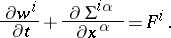where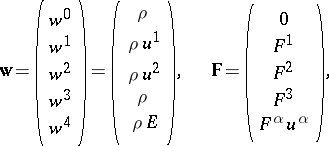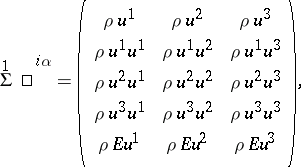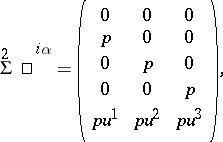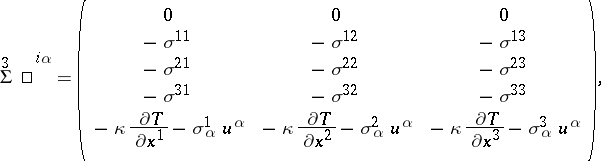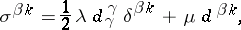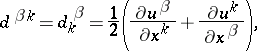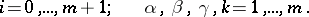Here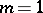for the one-dimensional case,for the two-dimensional case,for the three-dimensional case,is the density of the gas,is the pressure of the gas,is the velocity of the gas,is the temperature of the gas,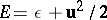is the total energy of a unit mass of the gas,is the specific internal energy,is the viscosity coefficient of compression,is the shear viscosity coefficient,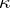is the coefficient of thermal conductivity, and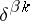is the Kronecker symbol.

Two basic classes of problems in gas dynamics are distinguished:

a) the Cauchy problem for a bounded or an unbounded domain (non-stationary problems of gas dynamics);

b) stationary boundary problems of gas dynamics for a bounded or an unbounded domain.

These problems may in turn be subdivided into several classes, depending on the physical properties of the flow of the gas. In accordance with this scheme one may distinguish between:) the flow of an ideal gas (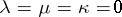);) the flow of a viscous incompressible gas (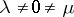,, whereis the compressibility coefficient,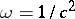, whereis the velocity of sound) described by the Navier–Stokes equations;) the flow of a viscous compressible heat-conducting gas (,).

Historically, the methods for solving problems of gas dynamics developed without any connection with these classes of problems. At present, a large number of finite-difference methods are available to obtain approximations of various orders. General principles for constructing numerical methods applicable to all problems of gas dynamics have been proposed, but not yet ultimately proved with mathematical rigour. These principles will now be summarized.

1) Representations of the generalized solution of the equations of an ideal gas as the limit of the respective solutions with physical () or artificial dissipative terms as these tend to zero. Artificial dissipative terms may be introduced directly into the equations of gas dynamics or may be implicitly defined by the structure of the finite-difference scheme itself (approximation viscosity).

2) Geometric, analytic and physical-process splittings (decompositions) of the integral conservation laws and of the differential equations themselves (the method of weak approximation, cf. Fractional steps, method of).

3) Representation of the stationary solution as the limit of the solutions of non-stationary problems (the adjustment method). Both hyperbolic and parabolic partial differential equations are used as auxiliary non-stationary problems.

4) Approximation of equations which are not of Cauchy–Kovalevskaya type by equations of Cauchy–Kovalevskaya type as an appropriate small parameter tends to zero (Navier–Stokes equations, filtration equations).

5) Construction of moving difference grids both of regular and of irregular types.

6) Subdivision of the approximation problems in a finite-difference scheme by the regular interior and boundary points.

7) Representation of a complicated system of linear or non-linear algebraic equations as recurrence relations (the method of approximate or exact factorization).

8) The method of extension of the boundary value problem beyond the boundary and its inclusion in a boundary value problem with a simple domain (the method of fictitious domains).

All these representations and methods have made it possible, in the final count, to reduce the algorithm of the solution of complicated problems of gas dynamics to algorithms for solving simple problems of a standard structure (modular analysis of algorithms). There is as yet no rigorous foundation for such an approach, but the approach has proved its worth in practice and is used with increasing frequency.

Numerical methods for solving problems in gas dynamics may be subdivided into two major classes: methods with explicit display of singularities (shock waves, contact boundaries, centred damped waves) and the so-called methods of continuous computation, in which the singularities are not explicit.

In the methods of the first class a generalized solution of the equations of gas dynamics is represented as a family of classical solutions, defined in certain domains covering the phase space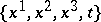and adjacent to one another by way of common boundaries (lines of discontinuity), adjacency conditions being satisfied (conditions of dynamic compatibility). In each domain it is possible to employ a finite-difference scheme which is suitable for classical solutions, while the adjacency conditions must be solved by a system of algebraic equations which are, as a rule, non-linear. One of the most widespread methods of discrete representation of classical solutions is the method of characteristics. This method is only used for solving problems of gas dynamics which can be described by hyperbolic equations; it is based on the fact that hyperbolic systems of equations have — for example, in the case of two unknown functions and two independent variables — a set of characteristics which form a characteristic grid constructed in the course of the computation. The method of characteristics appeared in gas dynamics a fairly-long time ago and was successfully employed in computing one-dimensional non-stationary flows with a small number of singularities, and also in the computation of two-dimensional stationary flows in a domain by hyperbolic equations. Modifications of this method are also used in the computations; here the computation is conducted by layers bounded by fixed lines. In the case of two independent variables (one-dimensional non-stationary problems or two-dimensional stationary problems, supersonic flow around bodies) the method of characteristics makes it possible to avoid interpolations and hence also the effects of smoothing and artificial viscosity. It gives an exact determination of the location of the shock waves inside the field of the possible flow, as a result of the intersection of the characteristics of one family. If the number of unknowns and independent variables is larger, the drawbacks of this method become evident: the approximation viscosity appears, and the algorithm becomes logically complicated when a large number of singularities appears. Another serious drawback of the method is the restriction on the step size of the grid, which is connected with the Courant stability criterion (cf. also Courant–Friedrichs–Lewy condition) and the fact that the conservation laws are not exactly fulfilled. Accordingly it is recommended to use the method of characteristics to solve problems in which the number of discontinuities is small. It has been proved that the solution by the method of characteristics converges to the solution of the initial differential problem if the flow is sufficiently smooth. As new computers will be developed capable of solving complicated logical problems, the method of characteristics will become more effective.

Another method which is used for solving these problems in gas dynamics is the method of integral relations (cf. Integral-relation method), which is applicable to equations of varying type. The method is based on the conservation laws and ultimately leads to solving ordinary differential equations.

The construction of finite-difference schemes for the problems of gas dynamics is based on the approximation of the conservation laws on a given moving or fixed grid, which yields a complicated system of explicit (explicit finite-difference schemes) or implicit (implicit finite-difference schemes) non-linear relations (cf. Hyperbolic partial differential equation, numerical methods).

Thus, for the equations of one-dimensional gas dynamics in Lagrangian coordinates it is possible to construct a general finite-difference scheme in the form: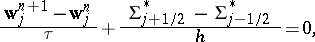(*)

where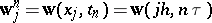. In order to close the relations (*) the magnitudes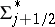,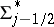must be connected with the magnitudes,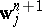. This may be done in various ways, which yield explicit finite-difference schemes if,are expressed in terms of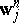, or else implicit finite-difference schemes if these sums are expressed in terms of,, and yield non-linear relations if one integral step is employed and linear relations if two or more fractional steps are utilized (the predictor-corrector method).

The solution of the equations obtained in this way may be greatly simplified if splitting methods — analytic (the predictor-corrector method), geometric or by physical processes — are employed. The geometric methods are employed in reducing multi-dimensional problems to problems of smaller dimensionality (the method of fractional steps or the splitting method). The splitting method yields economical, absolutely-approximating schemes in which the number of operations required to compute the desired functions at some point does not increase with the number of the points (cf. Difference scheme). One modification of the splitting method is the method of "particle-in-cells" , in which the splitting is not related to a reduction of the dimension of the operators.

This general method yields finite-difference schemes of continuous counting computation, both for an ideal gas () and for a dissipative process (). Such schemes, by introducing an approximation viscosity, smoothen the singularities in the transitional domains of width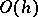and convert shock waves to shock transitions, and contact discontinuities to contact strips. The approximation viscosity of the finite-difference schemes comprises the dissipative properties of the equations of gas dynamics and those of the finite-difference scheme itself. The structure of the approximation viscosity is determined by the differential approximation of schemes which differ from the original system of equations by terms of order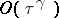, whereis the order of the approximation scheme. An important factor in many cases is the extent to which the finite-difference scheme and its differential approximation preserve the group properties of the initial system of differential equations. The preservation of the group properties by the finite-difference scheme is of great importance in practical computations, especially so in problems of gas dynamics in which, for example, the non-invariance of the first differential approximation with respect to the Galilean transformation brings about undesirable computational effects (instability, non-monotone profiles, etc.).

The known finite-difference schemes of continuous computations have a local accuracy on smooth solutions which is usually of order three at most and a global accuracy of order one at most (because of the low accuracy of the finite-difference scheme near singular points). Finite-difference schemes for equations of gas dynamics must satisfy not only the independent requirements of approximation and stability, but also several other practically-indispensable requirements — divergence, economy, complete conservation, etc. The idea of splitting makes it possible to construct economic finite-difference schemes for multi-dimensional problems. Divergence or conservation of a finite-difference scheme means that the finite-difference analogues of the fundamental laws of conservation (of mass, momentum and total energy) are satisfied for the finite-difference equations. The property of complete conservation requires a fulfillment of the finite-difference analogues of the conservation laws — not only the conservation of mass, momentum and total energy, but also of different types of energy (such as kinetic, potential or magnetic).

Below a few concrete finite-difference schemes of the form (*) are considered. If, in formula (*), one setsthe following explicit difference scheme of first-order approximation is obtained: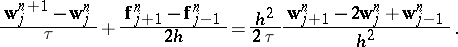Here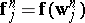. This scheme is a conditional approximation for(for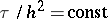it approximates the system of equations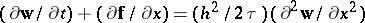), it is divergent and conditionally stable. The stability condition has the form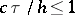whereis the velocity of sound.

Putting in formula (*)where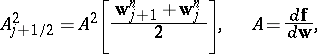one obtains an absolutely-approximating explicit finite-difference scheme of second-order approximation: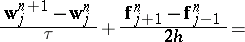This scheme is divergent and stable if.

The finite-difference scheme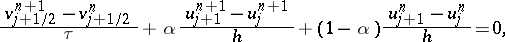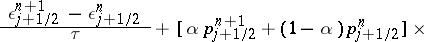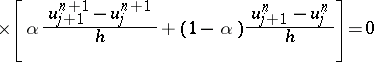is explicit if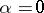; it is implicit if,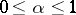; the approximation is of order two if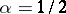and is of order one if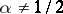; if, the scheme is totally conservative.

The finite-difference scheme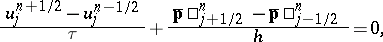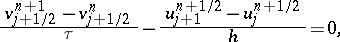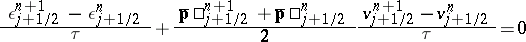gives a second-order approximation, is implicit, absolutely approximating and non-divergent. Here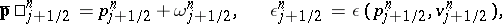The success of continuous-computation finite-difference schemes is due to the fact that, even though the approximation viscosity of the scheme determines the structure of the finite-difference shock transition, the conservative approximation preserves the velocity of the shock wave and reproduces conditions of dynamic compatibility.

However, these favourable features of continuous-computation finite-difference schemes lose their importance in cases in which the aim is to transmit accurately the structure of the shock transition (problems of radiative gas dynamics), the structure of the contact strip or the boundary layers. In the case of contact discontinuity a satisfactory approximation by a continuous-computation finite-difference scheme becomes impossible not only because of the strong extension of the contact strip, but also because of the appearance of Helmholtz and Taylor instabilities. In such cases the method of "particle-in-cell" can be successfully employed since, owing to the instability, strong boundary distortions can be transmitted.

In problems of flows of a viscous gas, for sufficiently large Reynolds numbers the effect of the approximation viscosity is larger than that of physical viscosity if the difference grid is not sufficiently fine. To diminish the effect of the approximation viscosity, the grid must be strongly refined near the body around which the flow is taking place. In practice, the flow problem is solved by two rather than by one method in this particular case, by subdividing the integration problem into two: the problem of flow of an ideal gas around the body in which the magnitude to be determined is, in particular, the rate of the flowon the boundaryof the body; and the problem of computing the viscous flow near the body, where the gradients of the magnitudes are large (the so-called boundary layer) with boundary conditions at infinity, where the specified velocity values are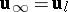. The formulation of the problem in the framework of boundary-layer theory is less rigorous than the integration of the Navier–Stokes equations, but is nevertheless most frequently employed in engineering computations for large Reynolds numbers.

An especially important class of problems in gas dynamics are problems of hydrodynamic instability and turbulence. In such a case the problems must be solved in eigen values for the hydrodynamic equations of a viscous liquid in variations (Orr–Sommerfeld equation) and complicated numerical models must be constructed to describe the non-linear instability and turbulence. Such problems belong to the most difficult ones in numerical mathematics and require the development of new models and powerful computers.